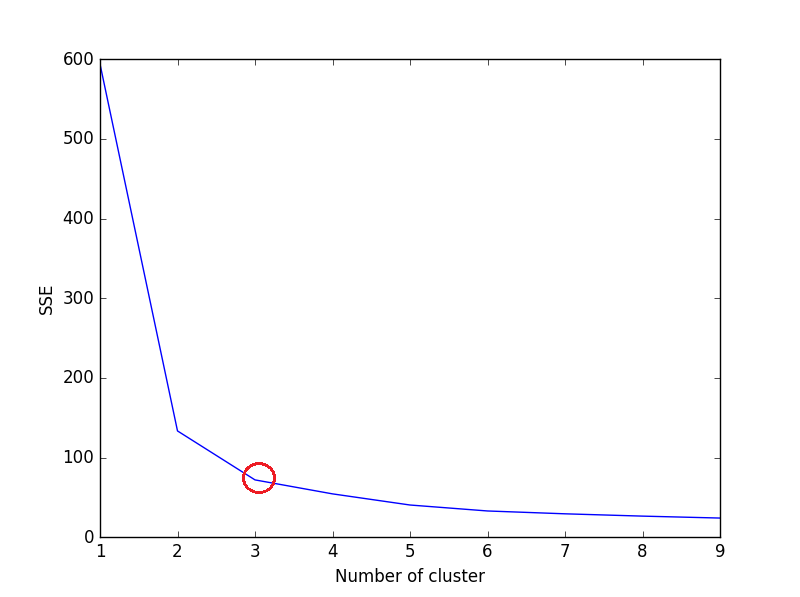2 views

Today I'm trying to learn something about K-means. I Have understood the algorithm and I know how it works. Now I'm looking for the right k... I found the elbow criterion as a method to detect the right k but I do not understand how to use it with scikit learn?! In scikit learn, I'm clustering things in this way

kmeans = KMeans(init='k-means++', n_clusters=n_clusters, n_init=10)

kmeans.fit(data)

So should I do this several times for n_clusters = 1...n and watch at the Error rate to get the right k? think this would be stupid and would take a lot of time?

by (33.1k points)

In your case, k-means clustering can be implemented using Elbow Criterion, if the true labels are known in advance.

Elbow Criterion Method:

The idea behind the elbow method is to implement k-means clustering on a given dataset for a range of values of k (num_clusters, e.g k=1 to 10), and for each value of k, calculate the sum of squared errors (SSE).

Elbow method plot a line graph of the SSE for each value of k. If the line graph looks like an arm - a red circle in the below line graph, the "elbow" on the arm is the value of optimal k (number of the cluster). K-means is used to minimize SSE. SSE tends to decrease toward 0 as we increase k and SSE is 0 when k is equal to the number of data points in the dataset, because then each data point is its own cluster, and there is no error between it and the center of its cluster.

So the goal is to choose a optimal value of k that still has a low SSE, and the elbow usually represents where we start to have diminishing returns by increasing k.

For Example:

from sklearn.cluster import KMeans

import matplotlib.pyplot as plt

sse = {}

for k in range(1, 10):

kmeans = KMeans(n_clusters=k, max_iter=1000).fit(data)

data["clusters"] = kmeans.labels_

print(data["clusters"])

sse[k] = kmeans.inertia_ # Inertia: Sum of distances of samples to their closest cluster center

plt.figure()

plt.plot(list(sse.keys()), list(sse.values()))

plt.xlabel("Number of cluster")

plt.ylabel("SSE")

plt.show()

Plot for above code: enter image description hereWe can see in the above plot, 3 is the optimal number of clusters (encircled red) for this dataset, which is indeed correct.